# Getting Started With GERGM

#### 2017-03-12

This vignette is designed to introduce you to the GERGM R package. GERGM stands for Generalized Exponential Random Graph Model. This class of models was developed to characterize the structure of networks with real-valued edges. GERGMs represent a generalization of ERGMs, which were developed to model the structure of networks with binary edge values, and many network statistics commonly included in ERGM specifications have identical formulations in the weighted case. The relevant papers detailing the model can be found at the links below:

• Bruce A. Desmarais, and Skyler J. Cranmer, (2012). “Statistical inference for valued-edge networks: the generalized exponential random graph model”. PloS One. [Available Here]
• James D. Wilson, Matthew J. Denny, Shankar Bhamidi, Skyler Cranmer, and Bruce Desmarais (2017). “Stochastic weighted graphs: Flexible model specification and simulation”. Social Networks, 49, 37–47. [Available Here]
• Matthew J. Denny (2016). “The Importance of Generative Models for Assessing Network Structure”. [Available Here]

## Installation

The easiest way to do this is to install the package from CRAN via the standard install.packages command:

install.packages("GERGM")

This will take care of some weird compilation issues that can arise, and is the best option for most people. If you want the most current development version of the package, you will need to start by making sure you have Hadley Wickham’s devtools package installed.

If you want to get the latest version from GitHub, start by checking out the Requirements for using C++ code with R section in the following tutorial: Using C++ and R code Together with Rcpp. You will likely need to install either Xcode or Rtools depending on whether you are using a Mac or Windows machine before you can install the GERGM package via GitHub, since it makes use of C++ code to speed up inference. That said, the development version often has additional functionality not found in the CRAN release.

install.packages("devtools")

Now we can install from Github using the following line:

devtools::install_github("matthewjdenny/GERGM")

Once the GERGM package is installed, you may access its functionality as you would any other package by calling:

library(GERGM)

If all went well, check out the vignette("getting_started") which will pull up this vignette!

## Basic Usage

We begin by loading in some example network data. In our case, these data are (logged) aggregate public and private lending volumes between 17 large countries from 2005. The data are included in the GERGM package and were used in the Wilson et. al. study listed at the beginning of this vignette. In addition to the network (a square matrix) we are also going to load in some node-level covariate data, and a network covariate: the normalized net exports between these countries in 2005. WE will make use of this data in fitting our example GERGM model.

The GERGM package provides a plot_network() function, which we can use to visualize the network as follows:

library(GERGM)
set.seed(12345)
data("lending_2005")
data("covariate_data_2005")
data("net_exports_2005")
plot_network(lending_2005)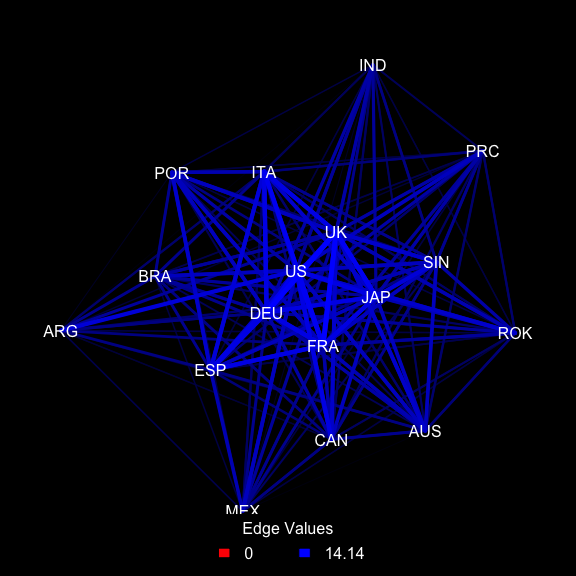Alternatively, if we prefer a white background, and no legend, we can select options for this as well. Typing ?plot_network into the console will pull up a manual for this function.

Having plotted the raw network data, we can now proceed to model it using the gergm() function. Detailed documentation for this function (along with a large number of advanced options) can be accessed by typing ?gergm into the console. We are going to focus on a simpler version of the application from the Wilson et. al. paper, that will highlight creating a formula object with node and network level covariates, as well as endogenous (network) effects. While this model will not provide a perfect fit to the data, it serves to illustrate a number of key concepts. If we look at the first couple of rows of the covariate_data_2005 object, we can see that it include information about each country’s log GDP and whether it was a member of the G8.

head(covariate_data_2005)
##              GDP  log_GDP  G8
## ARG 2.229108e+11 26.13004  No
## AUS 6.933386e+11 27.26478  No
## BRA 8.921068e+11 27.51685  No
## CAN 1.164144e+12 27.78301 Yes
## PRC 2.268594e+12 28.45018  No
## FRA 2.203679e+12 28.42115 Yes

To model this network, we are gong to include an edges term, which functions similarly to an intercept term in a regression model and parameterizes the density of the network. We are also going to include sender and receiver effects for a country’s GDP. These effects are designed to capture the effects of having a large economy on the amount of lending a borrowing a country does. We are also going to include a Nodemix term to capture the propensity for members and non-members of the G8 to lend to each other, compared to the base case of non-G8 to non-G8 member lending. The final covariate effect we are going to include in the model is a netcov, or network covariate term, capturing the effect of the structure of the international trade network on the international lending network. Finally, we are going to include one endogenous statistic in the model, to capture the degree of reciprocal lending in the network. For this endogenous statistic, we are also going to include an exponential down-weight. this means that when the value of the network statistic is calculated, it will then be raised to the power of (in this case) 0.8. This will have the effect of reducing its value, but more importantly of smoothing out statistic values as the GERGM parameter controlling the propensity for mutual dyads in the network carries. Practically, this can make it easier to get starting values for the mutual dyads parameter that are in the right ball park, aiding in the estimation process. The formula object is defined below:

formula <- lending_2005 ~ edges + mutual(alpha = 0.8) + sender("log_GDP") +
receiver("log_GDP") + nodemix("G8", base = "No") + netcov(net_exports_2005) 

Note that the terms used in GERGM formulas are analogous to those used in the ergm package, and are documented in greater detail in the ?gergm help file.

If you are interested in experimenting, try setting alpha = 1 and rerunning the model. You will see lots of error messages, and output indicating that your parameter estimates have zoomed off to infinity. If you are familiar with ERGMs (for binary network data), you may have heard of an issue these models can run into called “degeneracy”, which can make certain models impossible to estimate. In this particular example, as with all GERGM specifications we have tried so far, the GERGM does not seem to suffer from this issue. However, as the experiment described above can attest, GERGMs can still be difficult to estimate. This is primarily due to challenges in getting good starting values for our model parameters. The current implementation of the GERGM software does so using maximum pseudo likelihood (MPLE), which does a pretty good job in many cases. However, in some cases, such as the example here, it can be enough off the mark that the initial parameter guesses from MPLE simulate networks that look a lot different from the observed network. This can cause the optimizer in R (which is used to update our estimates of the model parameters) to zoom off to infinity.

If this happens to you, do not (immediately) panic! This usually means you are dealing with a tricky network, or a tricky specification (typically one with lots of endogenous statistics included). The first thing to do is try to use alpha weighting. A good rule of thumb is to set alpha = 0.8 for all of the endogenous statistics included in the model. Note that these currently include: out2stars, in2stars, ctriads, mutual, and ttriads (or just twostars and ttriads if your network is undirected). If this does not work, you can try cranking down the weights to around 0.5. If this still does not work, you will need to explore the theta_grid_optimization_list option in the gergm documentation, which should always work if given enough time (although this could be weeks, depending on how complex your model is). A fuller example is provided at the end of this vignette.

Having addressed the challenges that come with estimating a GERGM model, lets try and example!

test <- gergm(formula,
covariate_data = covariate_data_2005,
number_of_networks_to_simulate = 40000,
thin = 1/100,
proposal_variance = 0.05,
MCMC_burnin = 10000,
seed = 456,
convergence_tolerance = 0.5)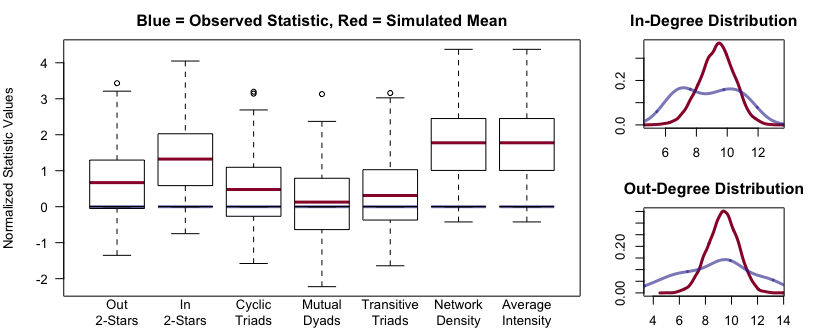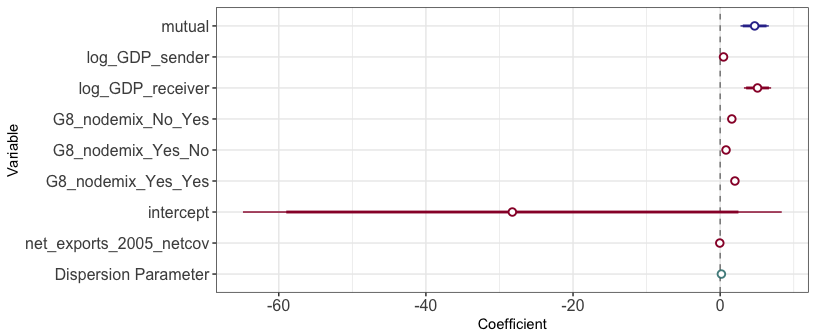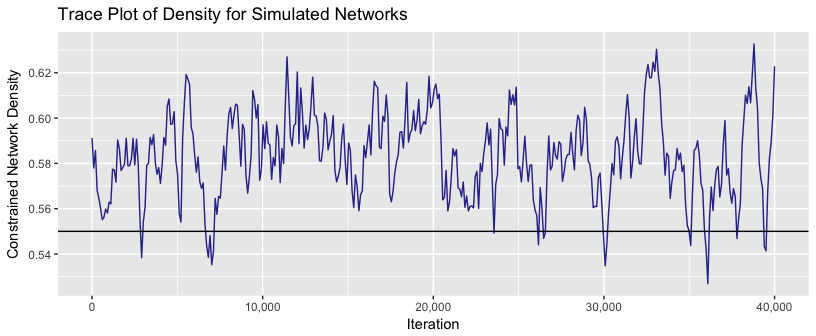The output displayed in this vignette only includes diagnostic plots, and not all of the information that would be spit out by the gergm() function if you were to run this code on your computer. All of that output is meant to help you track the estimation process (which can take days or weeks for larger networks), and diagnose issues with the estimation. Note that if you wish to tweak some of the parameters in the diagnostic and estimate plots, you may do so and regenerate the plots after estimation is complete using the following functions:

# Generate Estimate Plot
Estimate_Plot(test)
# Generate GOF Plot
GOF(test)
# Generate Trace Plot
Trace_Plot(test)

In particular, we might want to make a nicer looking estimate plot. We can do this using the following block of code, where we leave out the intercept estimate, and provide a custom list of parameter names to produce a publication quality plot:

Estimate_Plot(test,
coefficients_to_plot = "both",
"log(GDP) Sender",
"intercept",
"Normalized Net Exports",
"Dispersion Parameter"),
leave_out_coefficients = "intercept")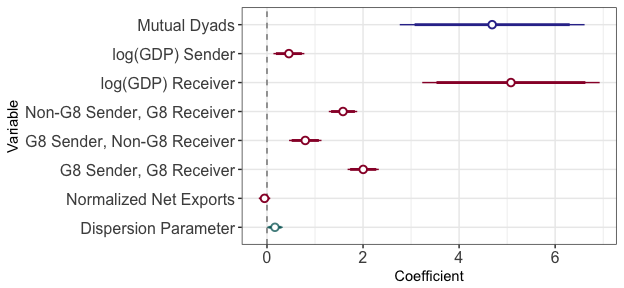In order to verify the claim made earlier in this vignette that the current model is not degenerate, just hard to fit, we can generate a hysteresis plot for this model using the hysteresis() function. This function simulates large numbers of networks at parameter values around the estimated parameter values and plots the mean network density at each of these values to examine whether the model becomes degenerate due to small deviations in the parameter estimates. See the following reference for details:

• Snijders, Tom AB, et al. “New specifications for exponential random graph models.” Sociological methodology 36.1 (2006): 99-153.

So long as we see a smooth upward sloping series of points, we have strong evidence that the specification is not degenerate.

# Generate Hysteresis plots for all structural parameter estimates
hysteresis_results <- hysteresis(test,
networks_to_simulate = 1000,
burnin = 300,
range = 8,
steps = 20,
simulation_method = "Metropolis",
proposal_variance = 0.05)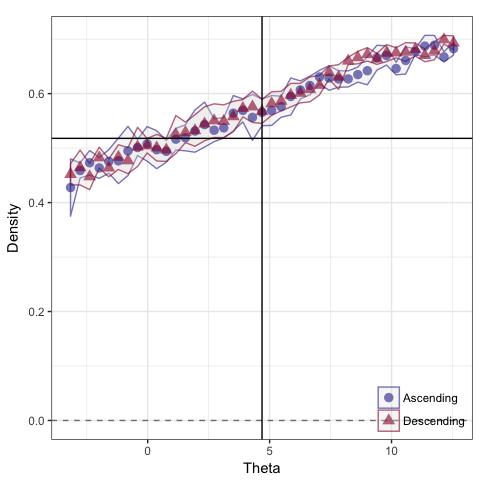As we can see this specification does not display signs of degeneracy, even though we needed to use exponential down-weighting in order to fit the model.

### Edge Prediction

Following on from the example above, we can also predict individual edge values, conditioning on the rest of the observed edges and estimated parameters. We can then calculate the mean edgewise mean squared error (MSE) for these predictions, and compare it against the MSE from a null model with no parameters included. First we generate the conditional edge predictions:

test2 <- conditional_edge_prediction(
GERGM_Object = test,
number_of_networks_to_simulate = 100,
thin = 1,
proposal_variance = 0.05,
MCMC_burnin = 100,
seed = 123)

Next we can calculate the MSE of these predictions and compare it to the null model predictions.

MSE_results <- conditional_edge_prediction_MSE(test2)
## Mean MSE for Predicted Edge Values: 8099.79
## Mean MSE for Max Ent Predicted Edge Values: 42927.12
## This represents a 81.13 percent reduction in the average edgewise MSE when using the GERGM model.

As we can see, this model does significantly better in terms of conditional edgewise predictive performance than the null model.

### Correlation Matrix Estimation Functionality

The GERGM development team has recently added functionality to estimate GERGMs on correlation matrices, as well as simulation functionality. The GERGM can therefore be used both to assess the structural properties of correlation matrices, but also as an infinitely flexible prior for correlation matrices. To access the this functionality, set the optional argument beta_correlation_model = TRUE. Covariate effects are modeled using a Beta regression and then the correlation matrix is transformed onto an unconstrained space of matrices on [0,1], where stadard GERGM modeling takes place. The resulting simulated networks can then be transformed back onto the correlation space. Correlation matrix estimation functionality is the same as with other undirected networks. More information on this model will be made available in mid 2017 with the publication of a paper that is now under review. Please contact the package maintainer with further questions.

  set.seed(12345)
# Function to generating a random positive-definite matrix with user-specified
# positive eigenvalues. If eigenvalues are not specified, they are generated
# from a uniform distribution.
Posdef <- function (n, ev = runif(n, 0, 10)) {
Z <- matrix(ncol=n, rnorm(n^2))
decomp <- qr(Z)
Q <- qr.Q(decomp)
R <- qr.R(decomp)
d <- diag(R)
ph <- d / abs(d)
O <- Q %*% diag(ph)
Z <- t(O) %*% diag(ev) %*% O
return(Z)
}

# Generate eignevalues
x <- rnorm(10)
# generate a positive definite matrix
pdmat <- Posdef(n = 10)
# transform to correlations
correlations <- pdmat / max(abs(pdmat))
diag(correlations) <- 1
net <- (correlations + t(correlations)) / 2

colnames(net) <- rownames(net) <- letters[1:10]

# correlation GERGM specification
formula <- net ~ edges + ttriads

# model should run in under a minute
test <- gergm(formula,
estimation_method = "Metropolis",
number_of_networks_to_simulate = 100000,
thin = 1/100,
proposal_variance = 0.2,
MCMC_burnin = 100000,
seed = 456,
convergence_tolerance = 0.5,
beta_correlation_model = TRUE)

## Common Problems and Errors

ERGMs and GERGM tend to be more challenging to fit to real world data than many other classes of models, due to the dependence between observations. We have sought to make the GERGM package as easy to use as possible, but it is likely that the user may still encounter some difficulties in fitting complex models, especially to large networks. Below, we outline several common challenges and errors a user may encounter and our advice for dealing with them. As a general note, always make sure the verbose option is set to TRUE when you are trying to diagnose any estimation problems, as this will give you much more information to work with.

• Time: The GERGM naturally has at least order N^2 time complexity (where N is the number of nodes in the network), which means that runtime increases exponentially as the network gets bigger. It may also take a large number of iterations for the model to reach the target distribution (a long burnin), and a large number of parameter updates for the model to converge. It is not uncommon for the model to run for a week or two, even on networks as small as 50 nodes. Runtime can also be dramatically increased by using some of the features we have built to aid in model fitting. To some degree, this is just part of the process, and the user should plan to run their analyses on a computer that will not be unplugged or restarted for a few weeks. Additionally, the user may use parallelization to help speed up this process. By setting parallel_statistic_calculation = TRUE and the cores argument to a number greater than one, a speedup in estimation may be achieved, particularly for larger networks (more than 50 nodes).
• Zero Acceptance Rate: The Metropolis Hastings estimation algorithm works by simulating a large sample of networks from the GERGM generative process to approximate the normalizing constant. However, if the proposal_variance is too large or the model is very complex, then the algorithm may never be able to accept a new network proposal, which will cause the estimation procedure to fail. This will be evidenced by the following line in the model output, near where an error occurs Metropolis Hastings Acceptance Rate (target = 0.25 ): 0. The error that actually stops estimation will usually be of the form: Error in if (abs(geweke_stat) > 1.7) { : because all network samples will be identical, so the convergence diagnostic statistic will be undefined. To deal with this error, try reducing the proposal variance by at least an order of magnitude. You can also set hyperparameter_optimization = TRUE, and the software will try to find an optimal proposal variance for you. However, you can speed things up by reducing the initial proposal variance yourself.
• Poor Initialization: While Wilson et al. (2017) show that the GERGM does not seem to exhibit classical likelihood degeneracy for most model specifications, if a particularly bad initialization for the parameters is selected by MPLE, then the Metropolis Hastings procedure may simulate networks such that the observed network is outside the convex hull of the simulated networks. If this happens, the optim function in R (which we use to update the parameter estimates) will tend to zoom off to infinity in an effort to correct the error, and the estimation procedure will crash. This is desirable behavior because such parameter estimates (on the order of 1,000,000,000) will be meaningless. You can tell that the model is displaying this sort of behavior if the final value after the line reading Optimizing theta estimates... in the R output is equal to something like -100000000000. One way to deal with this is to set hyperparameter_optimization = TRUE, which will try to automatically apply exponential down weights, optimize the proposal variance, and increase the number of iterations (but only if stop_for_degeneracy = FALSE, the default value) until the model is able to simulate networks where the observed network is inside the convex hull of simulated networks. Alternatively, the theta_grid_optimization_list option may be used, as in the example in the next section. This is a brute force approach which basically tries a number of parameter combinations around the MPLE estimates to find a combination that is good enough that the optimizer can do its work. The downside si that this requires a lot of computational power (and time), but this slowdown and be dramatically reduced by using multiple cores and parallelization. Adding more node level covariate effects to the model may also increase stability, but should not be done if those covariates are not theoretically justified.

While the above issues are not an exhaustive list, they are the most common ones we have encountered. Please check that your issue does not fall under one of the above categories (or that the solution described above does not work) before contacting the package maintainer.

## Bonus: A More Complex Model

Here we have included code to run the full model which appears in the Wilson et al. paper. It requires a 30 core machine to run as currently specified, and can take several days to weeks to run, depending on your computer setup. We include this more complex specification to highlight the flexibility the GERGM package gives users to deal with more difficult to model data. These more advanced features are covered in the ?gergm documentation, and will be the subject of future vignettes.

formula <- net ~  mutual(0.8) + ttriads(0.8) + out2stars(0.8) +
sender("log_GDP") + netcov(net_exports) +
receiver("log_GDP") + nodemix("G8", base = "No")

result <- gergm(formula,
covariate_data = covariate_data_2005,
number_of_networks_to_simulate = 400000,
thin = 1/100,
proposal_variance = 0.05,
MCMC_burnin = 200000,
seed = 456,
convergence_tolerance = 0.8,
hyperparameter_optimization = TRUE,
target_accept_rate = 0.25,
weighted_MPLE = TRUE,
theta_grid_optimization_list = list(grid_steps = 2,
step_size = 0.1,
cores = 30,
iteration_fraction = 1))